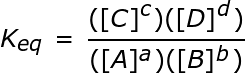# Equilibrium Constant (K) and Reaction Quotient (Q)

An error occurred trying to load this video.

Try refreshing the page, or contact customer support.

Coming up next: Using a RICE Table in Equilibrium Calculations

### You're on a roll. Keep up the good work!

Replay
Your next lesson will play in 10 seconds
• 0:05 A State of Equilibrium
• 0:25 Chemical Equilibrium
• 2:14 The Equilibrium Constant
• 3:39 The Reaction Quotient
• 4:51 Example Time
• 7:00 Lesson Summary
Save Save

Want to watch this again later?

Timeline
Autoplay
Autoplay
Speed Speed

#### Recommended Lessons and Courses for You

Lesson Transcript
Instructor: Artem Cheprasov

Artem has a doctor of veterinary medicine degree.

In this lesson, we will first define and explain the notion of a chemical equilibrium. Then, you'll learn about the equilibrium constant and reaction quotient. Finally, we'll round off the lesson with a couple of examples to solidify what you've learned!

## A State of Equilibrium

A balance scale with equal weights on each side, a stalemate during a tug of war, and an equal amount of supply and demand are all examples of a type of equilibrium, a kind of state of balance that's achieved due to opposing forces. Chemistry is subject to a notion of equilibrium as well, and we're going to go over it right now.

## Chemical Equilibrium

Imagine a simple chemical reaction with species A and B, denoted as follows:

A <-> B

The double arrow that's in the middle of A and B means that the reaction is going both ways. That is to say, the reactants are turning into the product species, while products are turning back into the reactant species.

Suppose that the A molecules start turning into the B molecules whenever the temperature rises above room temperature. Inasmuch, imagine placing a test tube with only A molecules into an oven that maintains a constant temperature above room temperature.

Given what I just mentioned, you would expect that some A molecules will start turning into B molecules. As time passes, more and more A molecules will turn into B molecules. However, suppose that there is also a backward tendency for B molecules to turn into A molecules for a large range of temperatures, including the one in the oven.

What will result is a back-and-forth movement between the concentrations of products and reactants, with the possibility of a chemical equilibrium eventually being established. A chemical equilibrium is a state in which the rate of the forward reaction is the same as the rate of the reverse reaction.

In our example, this means that after a certain period of time, the concentrations of species A and B will reach a stable state in which just as many A molecules will be turning into B molecules as B molecules turning into A molecules. In other words, the reaction will reach equilibrium, which could be maintained indefinitely if all factors, such as temperature, remain the same.

## The Equilibrium Constant

Knowing this, you can now understand the next part of equilibrium, as it relates to chemistry. There is a numerical value, called the equilibrium constant, which is a value that relates the ratio of the concentrations of products to reactants once the reaction has reached chemical equilibrium.

Going back to our example from the last section, we would be able to calculate this constant by knowing the concentrations of species A and B at chemical equilibrium.

But let's consider a more general reaction to drive the point of an equilibrium constant home. It reads as follows:

aA + bB <-> cC + dD

Here, a, b, c, and d are integers, and A, B, C, and D designate the chemical species in question. The square brackets designate taking the concentration of chemical species; that is, [A] is the concentration of A.

We can calculate the equilibrium constant, denoted as Keq, as follows:It is important to note that only species in the gas and aqueous state are included in the equilibrium constant calculation (pure solids and liquids are omitted).

## The Reaction Quotient

At this point, you may be wondering about what happens when the reaction is not in equilibrium. Meaning, is there a similar number that we can calculate to determine the state of the reaction in such a scenario? Why, yes there is!

To unlock this lesson you must be a Study.com Member.

### Register to view this lesson

Are you a student or a teacher?

#### See for yourself why 30 million people use Study.com

##### Become a Study.com member and start learning now.
Back
What teachers are saying about Study.com

### Earning College Credit

Did you know… We have over 200 college courses that prepare you to earn credit by exam that is accepted by over 1,500 colleges and universities. You can test out of the first two years of college and save thousands off your degree. Anyone can earn credit-by-exam regardless of age or education level.